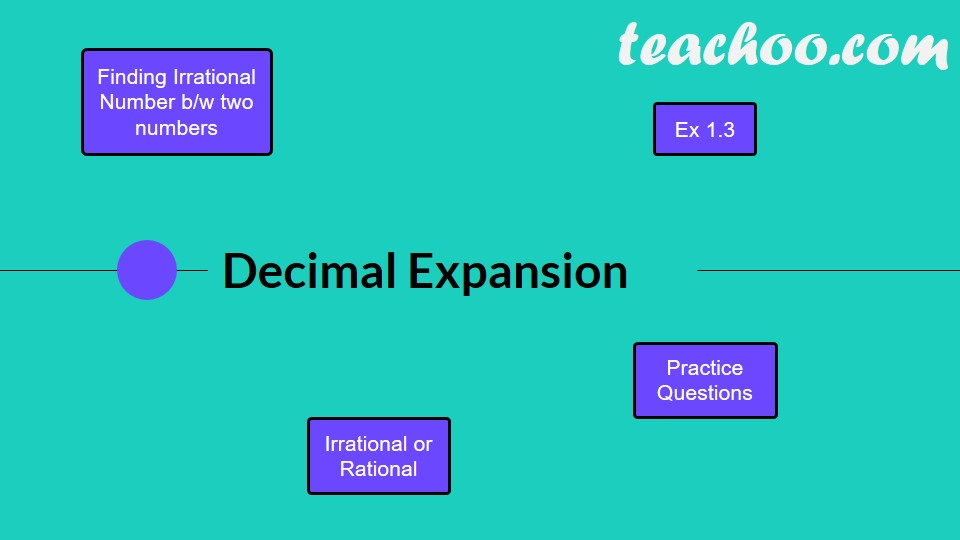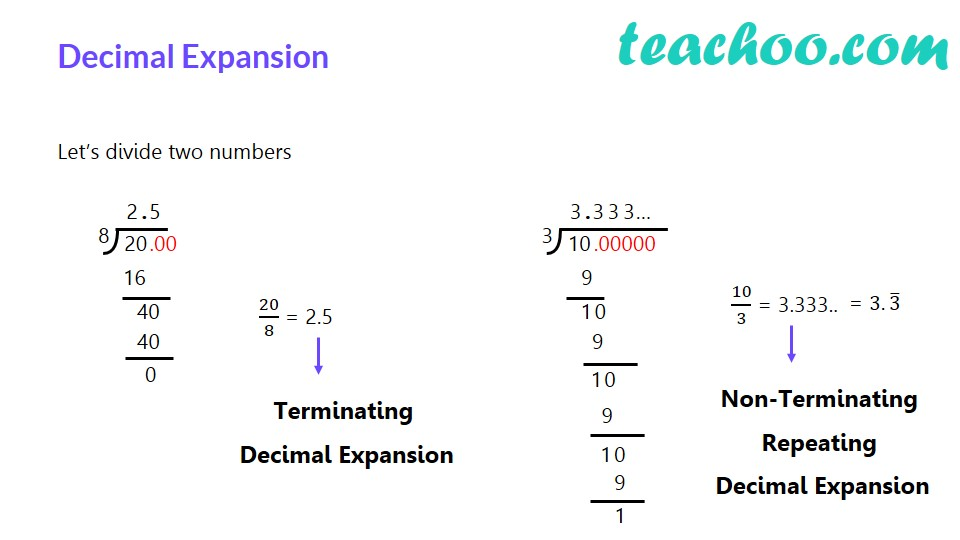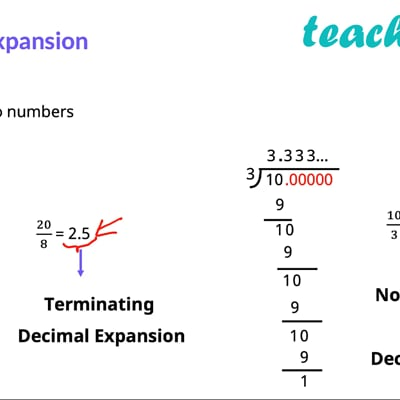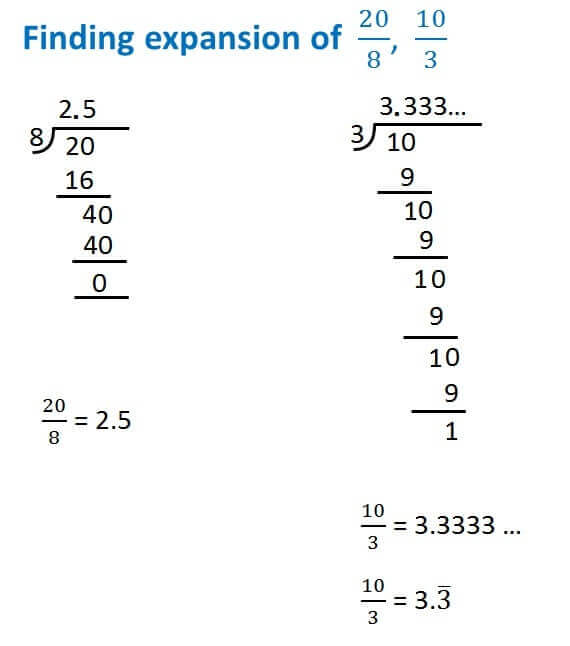Finding decimal expansion

Chapter 1 Class 9 Number Systems
Concept wiseThis video is only available for Teachoo black users

There are generally 3 types of decimal expansion

1. Terminating
2. Non-terminating Repeating
3. Non-terminating Non Repeating

Let us take an example to study itIn 20/8 = 2.5 ,

• the remainder is 0
• the decimal ends at 5.

So, that means the expansion is terminating.

In 10/3 = 3.3333 …,

• the expansion does not end i.e. non-terminating
• and 3 keeps on repeating

So, it is a non-terminating, repeating expansion

There are also some decimals like

1.4142135623…

• the expansion does not end i.e. non-terminating
• and no set of numbers repeat

So, it is a non-terminating, non-repeating expansion

Let us learn how to find decimal expansions of numbers.

Get live Maths 1-on-1 Classs - Class 6 to 12

### Transcript

Finding Irrational Number b/w two numbers Decimal Expansion Irrational or Rational Practice Questions Decimal Expansion Let’s divide two numbers 20/8 = 2.5 Terminating Decimal Expansion 10/3 = 3.333.. Non-Terminating Repeating Decimal Expansion